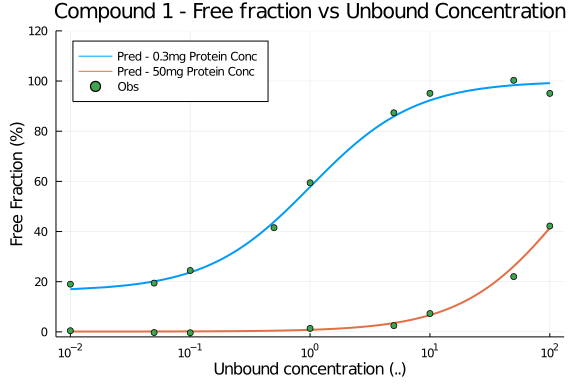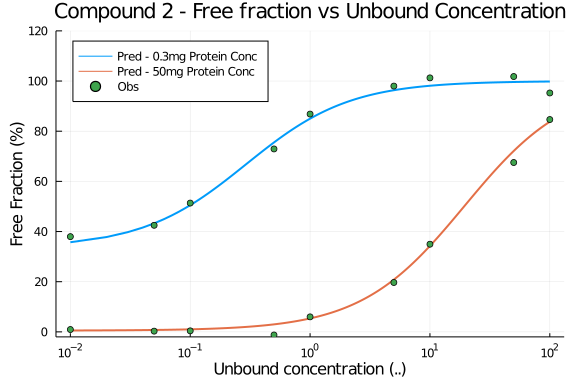# Exercise PK47 - Plasma Protein Binding Modeling

### Background

• Structrual Model - Plasma Protein Binding Model

• Number of Subjects - 4

• Number of Compounds - 2

### Learning Outcome

• To get an understanding of the determinants of the unbound concentration, free fraction and total concentration.

• How the modeling of plasma protein data is done

• To understand the relationship how the binding protein concentration effects the unbound drug concentration

### Objectives

• To analyze In vitro plasma protein data of two compounds

• To analyze binding data at two different binding concentration

• To show and understand the relationship between Cu and fu

• To understand the properties of binding site that can be modeled

### Libraries

call the necessary 'libraries' to get started

using Pumas
using Plots
using CSV
using StatsPlots
using Random


### Model

In this plasma protein binding model, we have included free drug concentration (dose) and total protein concentration (Pt) as a covariate and with the help of a simple non-linear fit we will estimate the parameters.

pk_47     = @model begin
@param begin
tvka  ∈ RealDomain(lower=0)
tvn   ∈ RealDomain(lower=0)
Ω     ∈ PDiagDomain(2)
σ_add ∈ RealDomain(lower=0)
end

@random begin
η     ~ MvNormal(Ω)
end

@covariates dose Pt

@pre begin
Ka    = tvka * exp(η)
n     = tvn * exp(η)
_dose = dose
_Pt   = Pt
end

@vars begin
fu    = (1-(1/(1+(_dose/(n*_Pt))+(1/(Ka*n*_Pt)))))*100
end

@derived begin
dv    = @. Normal(fu, σ_add)
end
end

PumasModel
Parameters: tvka, tvn, Ω, σ_add
Random effects: η
Covariates: dose, Pt
Dynamical variables:
Derived: dv, fu
Observed: dv, fu


### Parameters

The parameters are as given below. tv represents the typical value for parameters.

• tvka - Affinity constant between drug and protein

• tvn - Number of binding sites per molecule

## Compound 1
param1 = (tvka  = 6.09,
tvn   = 2.833,
Ω     = Diagonal([0.0,0.0,0.0]),
σ_add = 2.0398)

## Compound 2
param2 = (tvka  = 10.2353,
tvn   = 1.937,
Ω     = Diagonal([0.0,0.0,0.0]),
σ_add = 2.2774)


### Dosage Regimen

Since there is no dosage regimen we will create a dataframe with no events and read the file using read_pumas which will be used for simulation.

## Compound 1
df_sub1  = DataFrame(id=1, time=0:1:9999, dv=missing, dose=0.01:0.01:100, Pt=0.3)
df_sub2  = DataFrame(id=2, time=0:1:9999, dv=missing, dose=0.01:0.01:100, Pt=50)
df1      = vcat(df_sub1, df_sub2)
pop1     = read_pumas(df1, observations=[:dv], covariates=[:dose, :Pt], event_data=false)

## Compound 2
df_sub3  = DataFrame(id=3, time=0:1:9999, dv=missing, dose=0.01:0.01:100, Pt=0.1)
df_sub4  = DataFrame(id=4, time=0:1:9999, dv=missing, dose=0.01:0.01:100, Pt=10)
df2      = vcat(df_sub3, df_sub4)
pop2     = read_pumas(df2, observations=[:dv], covariates=[:dose, :Pt], event_data=false)


### Simulation

We will now simulate the experimental dat for Compound 1 at two different levels of protein concentration(Pt) 0.3 and 50.

##Compound 1
Random.seed!(123)
sim_1    = simobs(pk_47, pop1, param1)
df1_cmp1 = DataFrame(sim_1)

## Compound 2
Random.seed!(123)
sim_2    = simobs(pk_47, pop2, param2)
df2_cmp2 = DataFrame(sim_2)


### Dataframe and Plotting

Compound 1

id1 = filter(x -> x.id == "1", df1_cmp1)
id2 = filter(x -> x.id == "2", df1_cmp1)
df1_dv_cmp1 = filter(x -> x.dose in [0.01,0.05,0.1,0.5,1,5,10,50,100], df1_cmp1)

@df id1 plot(:dose , :fu, xaxis=:log, linewidth=2,
title = "Compound 1 - Free fraction vs Unbound Concentration", label="Pred - 0.3mg Protein Conc",
xlabel="Unbound concentration (..)", ylabel="Free Fraction (%)", legend=:topleft,
ylims=(-2,120), yticks=[0,20,40,60,80,100,120])
@df id2 plot!(:dose , :fu, xaxis=:log, label="Pred - 50mg Protein Conc", linewidth=2)
@df df1_dv_cmp1 scatter!(:dose, :dv, label="Obs")Compound 2

id3 = filter(x -> x.id == "3", df2_cmp2)
id4 = filter(x -> x.id == "4", df2_cmp2)
df2_dv_cmp2 = filter(x -> x.dose in [0.01,0.05,0.1,0.5,1,5,10,50,100], df2_cmp2)

@df id3 plot(:dose , :fu, xaxis=:log, linewidth=2,
title = "Compound 2 - Free fraction vs Unbound Concentration", label="Pred - 0.3mg Protein Conc", legend=:topleft,
xlabel="Unbound concentration (..)", ylabel="Free Fraction (%)",
ylims=(-2,120), yticks=[0,20,40,60,80,100,120])
@df id4 plot!(:dose , :fu, xaxis=:log, label="Pred - 50mg Protein Conc", linewidth=2)
@df df2_dv_cmp2 scatter!(:dose, :dv, label="Obs")